Topics: Coronavirus | AI | Data Science | Deep Learning | Machine Learning | Python | R | Statistics

KDnuggets Home » News » 2019 » Apr » Tutorials, Overviews » How Machines Make Sense of Big Data: an Introduction to Clustering Algorithms ( 19:n15 )

# How Machines Make Sense of Big Data: an Introduction to Clustering Algorithms

We outline three different clustering algorithms - k-means clustering, hierarchical clustering and Graph Community Detection - providing an explanation on when to use each, how they work and a worked example.comments

By Peter Gleeson, Revolut.Take a look at the image below. It’s a collection of bugs and creepy-crawlies of different shapes and sizes. Take a moment to categorize them by similarity into a number of groups.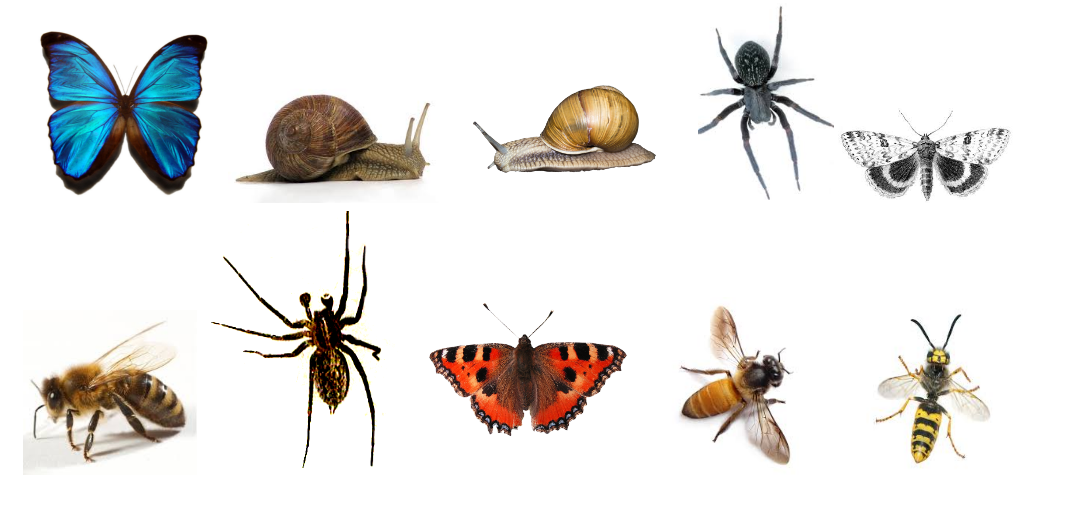Images via Google Image Search, labelled for reuse

Done? While there’s not necessarily a “correct” answer here, it’s most likely you split the bugs into four clusters. The spiders in one cluster, the pair of snails in another, the butterflies and moth into one, and the trio of wasps and bees into one more.

That wasn’t too bad, was it? You could probably do the same with twice as many bugs, right? If you had a bit of time to spare — or a passion for entomology — you could probably even do the same with a hundred bugs.

For a machine though, grouping ten objects into however many meaningful clusters is no small task, thanks to a mind-bending branch of maths called combinatorics, which tells us that are 115,975 different possible ways you could have grouped those ten insects together. Had there been twenty bugs, there would have been over fifty trillion possible ways of clustering them.

With a hundred bugs — there’d be many times more solutions than there are particles in the known universe. How many times more? By my calculation, approximately five hundred million billion billion times more. In fact, there are more than four million billion googol solutions (what’s a googol?). For just a hundred objects.

Almost all of those solutions would be meaningless — yet from that unimaginable number of possible choices, you pretty quickly found one of the very few that clustered the bugs in a useful way.

Us humans take it for granted how good we are categorizing and making sense of large volumes of data pretty quickly. Whether it’s a paragraph of text, or images on a screen, or a sequence of objects — humans are generally fairly efficient at making sense of whatever data the world throws at us.

Given that a key aspect of developing A.I. and Machine Learning is getting machines to quickly make sense of large sets of input data, what shortcuts are there available? Here, you can read about three clustering algorithms that machines can use to quickly make sense of large datasets. This is by no means an exhaustive list — there are other algorithms out there — but they represent a good place to start!

You’ll find for each a quick summary of when you might use them, a brief overview of how they work, and a more detailed, step-by-step worked example. I believe it helps to understand an algorithm by actually carrying out yourself. If you’re really keen, you’ll find the best way to do this is with pen and paper. Go ahead — nobody will judge!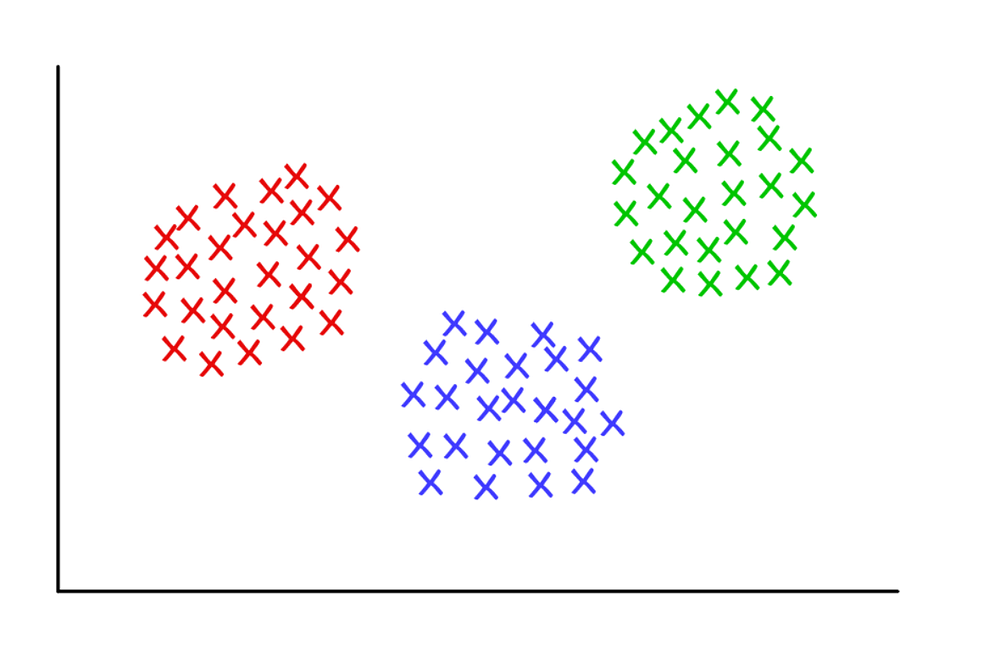Three suspiciously neat clusters, with K = 3

### K-means clustering

#### Use when:

…you have an idea of how many groups you’re expecting to find a priori.

#### How it works:

The algorithm randomly assigns each observation into one of categories, then calculates the mean of each category. Next, it reassigns each observation to the category with the closest mean before recalculating the means. This step repeats over and over until no more reassignments are necessary.

#### Worked Example:

Take a group of 12 football (or ‘soccer’) players who have each scored a certain number of goals this season (say in the range 3–30). Let’s divide them into separate clusters — say three.

Step 1 requires us to randomly split the players into three groups and calculate the means of each.

```Group 1
Player A (5 goals), Player B (20 goals), Player C (11 goals)
Group Mean = (5 + 20 + 11) / 3 = 12
Group 2
Player D (5 goals), Player E (3 goals), Player F (19 goals)
Group Mean = 9
Group 3
Player G (30 goals), Player H (3 goals), Player I (15 goals)
Group Mean = 16
```

Step 2: For each player, reassign them to the group with the closest mean. E.g., Player A (5 goals) is assigned to Group 2 (mean = 9). Then recalculate the group means.

```Group 1 (Old Mean = 12)
Player C (11 goals)
New Mean = 11
Group 2 (Old Mean = 9)
Player A (5 goals), Player D (5 goals), Player E (3 goals), Player H (3 goals)
New Mean = 4
Group 3 (Old Mean = 16)
Player G (30 goals), Player I (15 goals), Player B (20 goals), Player F (19 goals)
New Mean = 21
```

Repeat Step 2 over and over until the group means no longer change. For this somewhat contrived example, this happens on the next iteration. Stop! You have now formed three clusters from the dataset!

```Group 1 (Old Mean = 11)
Player C (11 goals), Player I (15 goals)
Final Mean = 13
Group 2 (Old Mean = 4)
Player A (5 goals), Player D (5 goals), Player E (3 goals), Player H (3 goals)
Final Mean = 4
Group 3 (Old Mean = 21)
Player G (30 goals), Player B (20 goals), Player F (19 goals)
Final Mean = 23
```

With this example, the clusters could correspond to the players’ positions on the field — such as defenders, midfielders and attackers. K-means works here because we could have reasonably expected the data to fall naturally into these three categories.

In this way, given data on a range of performance statistics, a machine could do a reasonable job of estimating the positions of players from any team sport — useful for sports analytics, and indeed any other purpose where classification of a dataset into predefined groups can provide relevant insights.

#### Finer details:

There are several variations on the algorithm described here. The initial method of ‘seeding’ the clusters can be done in one of several ways. Here, we randomly assigned every player into a group, then calculated the group means. This causes the initial group means to tend towards being similar to one another, which ensures greater repeatability.

An alternative is to seed the clusters with just one player each, then start assigning players to the nearest cluster. The returned clusters are more sensitive to the initial seeding step, reducing repeatability in highly variable datasets. However, this approach may reduce the number of iterations required to complete the algorithm, as the groups will take less time to diverge.

An obvious limitation to K-means clustering is that you have to provide a priori assumptions about how many clusters you’re expecting to find. There are methods to assess the fit of a particular set of clusters. For example, the Within-Cluster Sum-of-Squares is a measure of the variance within each cluster. The ‘better’ the clusters, the lower the overall WCSS.

### Hierarchical clustering

#### Use when:

…you wish to uncover the underlying relationships between your observations.

#### How it works:

A distance matrix is computed, where the value of cell (i, j) is a distance metric between observations and j. Then, pair the closest two observations and calculate their average. Form a new distance matrix, merging the paired observations into a single object. From this distance matrix, pair up the closest two observations and calculate their average. Repeat until all observations are grouped together.

#### Worked example:

Here’s a super-simplified dataset about a selection of whale and dolphin species. As a trained biologist, I can assure you we normally use much more detailed datasets for things like reconstructing phylogeny. For now though, we’ll just look at the typical body lengths for these six species. We’ll be using just two repeated steps.

```Species          Initials  Length(m)
Bottlenose Dolphin     BD        3.0
Risso's Dolphin        RD        3.6
Pilot Whale            PW        6.5
Killer Whale           KW        7.5
Humpback Whale         HW       15.0
Fin Whale              FW       20.0
```

Step 1: compute a distance matrix between each species. Here, we’ll use the Euclidean distance — how far apart are the data points? Read this exactly as you would a distance chart in a road atlas. The difference in length between any pair of species can be looked up by reading the value at the intersection of the relevant row and column.

```   BD   RD   PW   KW   HW
RD  0.6
PW  3.5  2.9
KW  4.5  3.9  1.0
HW 12.0 11.4  8.5  7.5
FW 17.0 16.4 13.5 12.5  5.0
```

Step 2: Pair up the two closest species. Here, this will be the Bottlenose & Risso’s Dolphins, with an average length of 3.3m.

Repeat Step 1 by recalculating the distance matrix, but this time merge the Bottlenose & Risso’s Dolphins into a single object with length 3.3m.

```[BD, RD]   PW   KW   HW
PW       3.2
KW       4.2   1.0
HW      11.7   8.5  7.5
FW      16.7  13.5 12.5  5.0
```

Next, repeat Step 2 with this new distance matrix. Here, the smallest distance is between the Pilot & Killer Whales, so we pair them up and take their average — which gives us 7.0m.

Then, we repeat Step 1 — recalculate the distance matrix, but now we’ve merged the Pilot & Killer Whales into a single object of length 7.0m.

```[BD, RD] [PW, KW]   HW
[PW, KW]      3.7
HW           11.7      8.0
FW           16.7     13.0   5.0
```

Next, we repeat Step 2 with this distance matrix. The smallest distance (3.7m) is between the two merged objects — so now we merge them into an even bigger object, and take the average (which is 5.2m).

Then, we repeat Step 1 and compute a new distance matrix, having merged the Bottlenose & Risso’s Dolphins with the Pilot & Killer Whales.

```   [[BD, RD] , [PW, KW]]    HW
HW                   9.8
FW                  14.8   5.0```

Next, we repeat Step 2. The smallest distance (5.0m) is between the Humpback & Fin Whales, so we merge them into a single object, and take the average (17.5m).

Then, it’s back to Step 1 — compute the distance matrix, having merged the Humpback & Fin Whales.

```[[BD, RD] , [PW, KW]]
[HW, FW]                  12.3
```

Finally, we repeat Step 2 — there is only one distance (12.3m) in this matrix, so we pair everything into one big object, and now we can stop! Let’s look at the final merged object:

```[[[BD, RD],[PW, KW]],[HW, FW]]
```

It has a nested structure (think JSON), which allows it to be drawn up as a tree-like graph, or dendrogram. It reads in much the same way a family tree might. The nearer two observations are on the tree, the more similar or closely-related they are taken to be.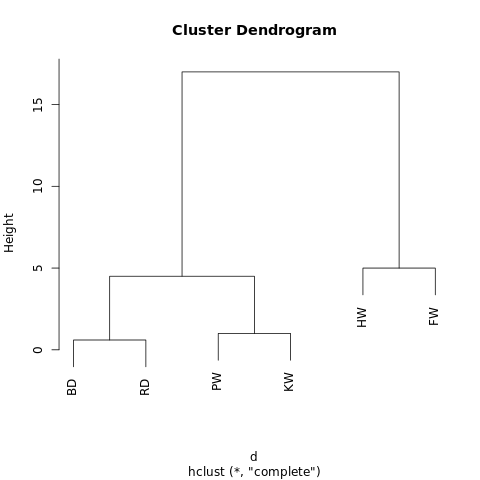A no-frills dendrogram generated at R-Fiddle.org

The structure of the dendrogram gives us insight into how our dataset is structured. In our example, we see two main branches, with Humpback Whale and Fin Whale on one side, and the Bottlenose Dolphin/Risso’s Dolphin and Pilot Whale/Killer Whale on the other.

In evolutionary biology, much larger datasets with many more specimens and measurements are used in this way to infer taxonomic relationships between them. Outside of biology, hierarchical clustering has applications in Data Mining and Machine Learning contexts.

The cool thing is that this approach requires no assumptions about the number of clusters you’re looking for. You can split the returned dendrogram into clusters by “cutting” the tree at a given height. This height can be chosen in a number of ways, depending on the resolution at which you wish to cluster the data.

For instance, looking at the dendrogram above, if we draw a horizontal line at height = 10, we’d intersect the two main branches, splitting the dendrogram into two sub-graphs. If we cut at height = 2, we’d be splitting the dendrogram into three clusters.

#### Finer details:

There are essentially three aspects in which hierarchical clustering algorithms can vary to the one given here.

Most fundamental is the approach — here, we have used an agglomerativeprocess, whereby we start with individual data points and iteratively cluster them together until we’re left with one large cluster. An alternative (but more computationally intensive) approach is to start with one giant cluster, and then proceed to divide the data into smaller and smaller clusters until you’re left with isolated data points.

There are also a range of methods that can be used to calculate the distance matrices. For many purposes, the Euclidean distance (think Pythagoras’ Theorem) will suffice, but there are alternatives that may be more applicable in some circumstances.

Finally, the linkage criterion can also vary. Clusters are linked according to how close they are to one another, but the way in which we define ‘close’ is flexible. In the example above, we measured the distances between the means (or ‘centroids’) of each group and paired up the nearest groups. However, you may want to use a different definition.

For example, each cluster is made up of several discrete points. We could define the distance between two clusters to be the minimum (or maximum) distance between any of their points — as illustrated in the figure below. There are still other ways of defining the linkage criterion, which may be suitable in different contexts.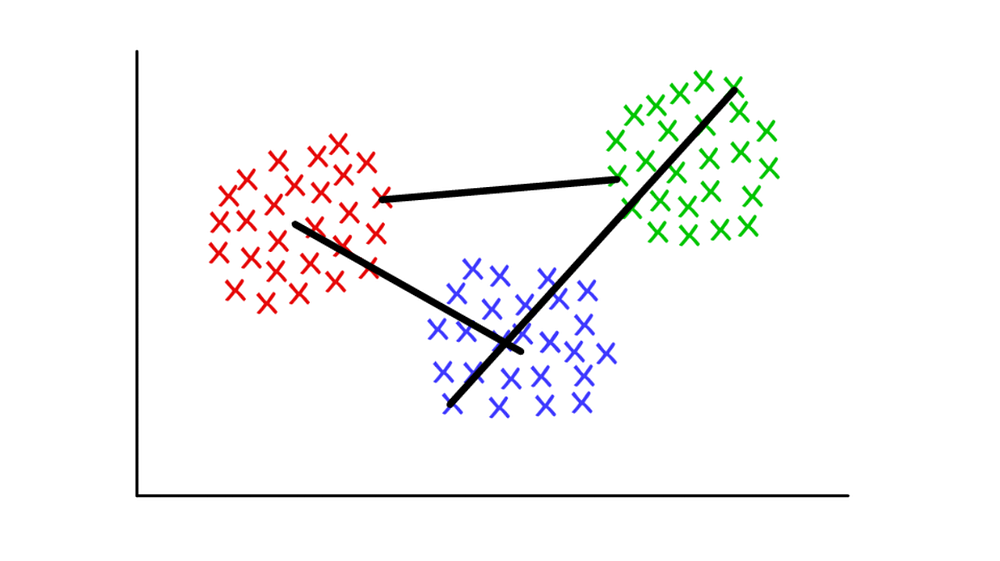### Graph Community Detection

#### Use when:

…you have data that can be represented as a network, or ‘graph’.

#### How it works:

graph community is very generally defined as a subset of vertices which are more connected to each other than with the rest of the network. Various algorithms exist to identify communities, based upon more specific definitions. Algorithms include, but are not limited to, Edge Betweenness, Modularity-Maximsation, Walktrap, Clique Percolation, Leading Eigenvector…

#### Worked example:

Graph theory, or the mathematical study of networks, is a fascinating branch of mathematics that lets us model complex systems as an abstract collection of ‘dots’ (or vertices) connected by ‘lines’ (or edges).

Perhaps the most intuitive case-studies are social networks. Here, the vertices represent people, and edges connect vertices who are friends/followers. However, any system can be modelled as a network if you can justify a method to meaningfully connect different components. Among the more innovative applications of graph theory to clustering include feature extraction from image data, and analysing gene regulatory networks.

As an entry-level example, take a look at this quickly put-together graph. It shows the eight websites I most recently visited, linked according to whether their respective Wikipedia articles link out to one another. You could assemble this data manually, but for larger-scale projects, it’s much quicker to write a Python script to do the same. Here’s one I wrote earlier.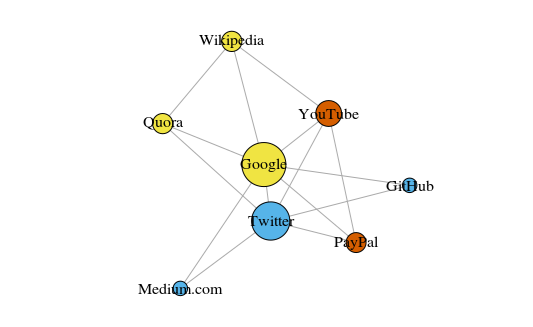Graph plotted with ‘igraph’ package for R version 3.3.3

The vertices are colored according to their community membership, and sized according to their centrality. See how Google and Twitter are the most central?

Also, the clusters make pretty good sense in the real-world (always an important performance indicator). The yellow vertices are generally reference/look-up sites; the blue vertices are all used for online publishing (of articles, tweets, or code); and the red vertices include YouTube, which was of course founded by former PayPal employees. Not bad deductions for a machine!

Aside from being a useful way to visualize large systems, the real power of networks comes from their mathematical analysis. Let’s start by translating our nice picture of the network into a more mathematical format. Below is the adjacency matrix of the network.

```GH Gl  M  P  Q  T  W  Y
GitHub    0  1  0  0  0  1  0  0
Google    1  0  1  1  1  1  1  1
Medium    0  1  0  0  0  1  0  0
PayPal    0  1  0  0  0  1  0  1
Quora     0  1  0  0  0  1  1  0
Twitter   1  1  1  1  1  0  0  1
Wikipedia 0  1  0  0  1  0  0  0
YouTube   0  1  0  1  0  1  0  0
```

The value at the intersection of each row and column records whether there is an edge between that pair of vertices. For instance, there is an edge between Medium and Twitter (surprise, surprise!), so the value where their rows/columns intersect is 1. Similarly, there is no edge between Medium and PayPal, so the intersection of their rows/columns returns 0.

Encoded within the adjacency matrix are all the properties of this network — it gives us the key to start unlocking all manner of valuable insights. For a start, summing any column (or row) gives you the degree of each vertex — i.e., how many others it is connected to. This is commonly denoted with the letter k.

Likewise, summing the degrees of every vertex and dividing by two gives you L, the number of edges (or ‘links’) in the network. The number of rows/columns gives us N, the number of vertices (or ‘nodes’) in the network.

Knowing just kL, N and the value of each cell in the adjacency matrix A lets us calculate the modularity of any given clustering of the network.

Say we’ve clustered the network into a number of communities. We can use the modularity score to assess the ‘quality’ of this clustering. A higher score will show we’ve split the network into ‘accurate’ communities, whereas a low score suggests our clusters are more random than insightful. The image below illustrates this.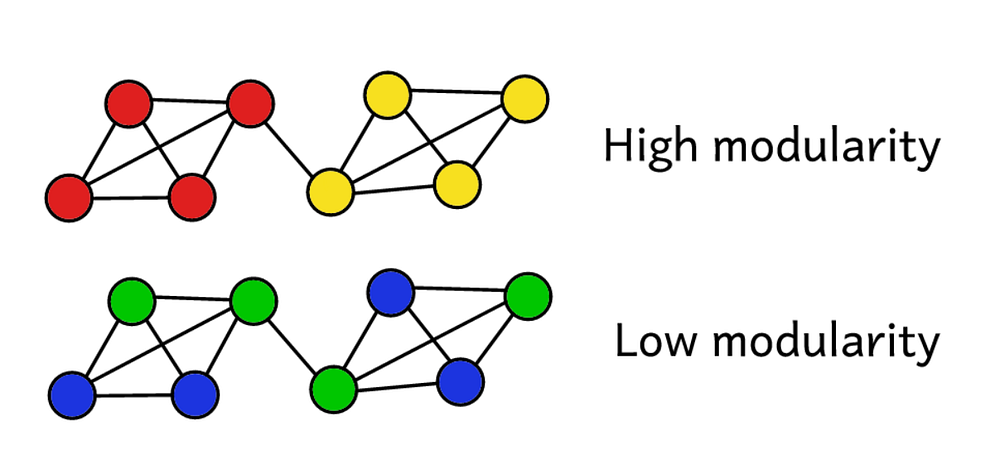Modularity serves as a measure of the ‘quality’ of a partition.

Modularity can be calculated using the formula below: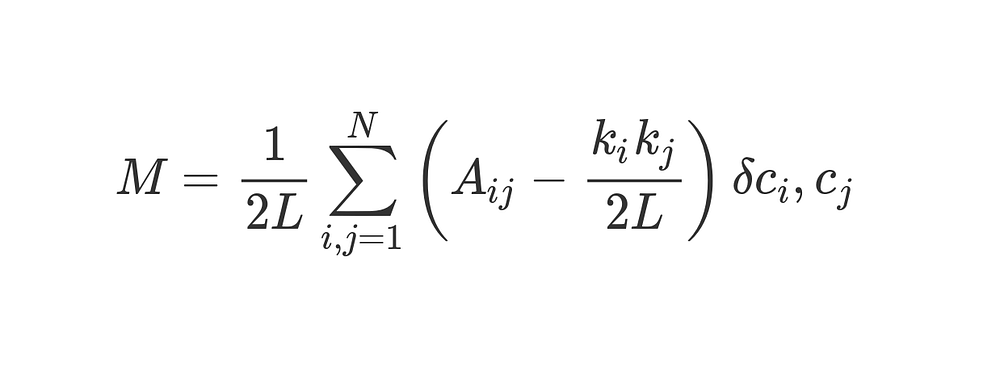That’s a fair amount of math, but we can break it down bit by bit and it’ll make more sense.

M is of course what we’re calculating — modularity.

1/2L tells us to divide everything that follows by 2L, i.e., twice the number of edges in the network. So far, so good.

The Σ symbol tells us we’re summing up everything to the right, and lets us iterate over every row and column in the adjacency matrix A. For those unfamiliar with sum notation, the i, j = 1 and the N work much like nested for-loops in programming. In Python, you’d write it as follows:

```sum = 0
for i in range(1,N):
for j in range(1,N):
ans = #stuff with i and j as indices
sum += ans
```

So what is #stuff with i and j in more detail?

Well, the bit in brackets tells us to subtract ( k_i k_j ) / 2L from A_ij.

A_ij is simply the value in the adjacency matrix at row i, column j.

The values of k_i and k_j are the degrees of each vertex — found by adding up the entries in row and column respectively. Multiplying these together and dividing by 2gives us the expected number of edges between vertices and if the network were randomly shuffled up.

Overall, the term in the brackets reveals the difference between the network’s real structure and the expected structure it would have if randomly reassembled. Playing around with the values shows that it returns its highest value when A_ij = 1, and ( k_i k_j ) / 2L is low. This means we see a higher value if there is an ‘unexpected’ edge between vertices and j.

Finally, we multiply the bracketed term by whatever the last few symbols refer to.

The 𝛿c_ic_j is the fancy-sounding but totally harmless Kronecker-delta function. Here it is, explained in Python:

```def Kronecker_Delta(ci, cj):
if ci == cj:
return 1
else:
return 0

Kronecker_Delta("A","A")    #returns 1
Kronecker_Delta("A","B")    #returns 0
```

Yes — it really is that simple. The Kronecker-delta function takes two arguments, and returns 1 if they are identical, otherwise, zero.

This means that if vertices i and have been put in the same cluster, then 𝛿c_ic_j = 1. Otherwise, if they are in different clusters, the function returns zero.

As we are multiplying the bracketed term by this Kronecker-delta function, we find that for the nested sum Σ, the outcome is highest when there are lots of ‘unexpected’ edges connecting vertices assigned to the same cluster. As such, modularity is a measure of how well-clustered the graph is into separate communities.

Dividing by 2L bounds the upper value of modularity at 1. Modularity scores near to or below zero indicate the current clustering of the network is really no use. The higher the modularity, the better the clustering of the network into separate communities. By maximising modularity, we can find the best way of clustering the network.

Notice that we have to pre-define how the graph is clustered to find out how ‘good’ that clustering actually is. Unfortunately, employing brute force to try out every possible way of clustering the graph to find which has the highest modularity score would be computationally impossible beyond a very limited sample size.

Combinatorics tells us that for a network of just eight vertices, there are 4140 different ways of clustering them. A network twice the size would have over ten billion possible ways of clustering the vertices. Doubling the network again (to a very modest 32 vertices) would give 128 septillion possible ways, and a network of eighty vertices would be cluster-able in more ways than there are atoms in the observable universe.

Instead, we have to turn to a heuristic method that does a reasonably good job at estimating the clusters that will produce the highest modularity score, without trying out every single possibility. This is an algorithm called Fast-Greedy Modularity-Maximization, and it’s somewhat analogous to the agglomerative hierarchical clustering algorithm describe above. Instead of merging according to distance, ‘Mod-Max’ merges communities according to changes in modularity. Here’s how it goes:

Begin by initially assigning every vertex to its own community, and calculating the modularity of the whole network, M.

Step 1 requires that for each community pair linked by at least a single edge, the algorithm calculates the resultant change in modularity Δif the two communities were merged into one.

Step 2 then takes the pair of communities that produce the biggest increase in ΔM, which are then merged. Calculate the new modularity for this clustering, and keep a record of it.

Repeat steps 1 and 2 — each time merging the pair of communities for which doing so produces the biggest gain in ΔM, then recording the new clustering pattern and its associated modularity score M.

Stop when all the vertices are grouped into one giant cluster. Now the algorithm checks the records it kept as it went along, and identifies the clustering pattern that returned the highest value of M. This is the returned community structure.

#### Finer details:

Whew! That was computationally intensive, at least for us humans. Graph theory is a rich source of computationally challenging, often NP-hard problems — yet it also has incredible potential to provide valuable insights into complex systems and datasets. Just ask Larry Page, whose eponymous PageRank algorithm — which helped propel Google from start-up to basically world domination in less than a generation — was based entirely in graph theory.

Community detection is a major focus of current research in graph theory, and there are plenty of alternatives to Modularity-Maximization, which while useful, does have some drawbacks.

For a start, its agglomerative approach often sees small, well-defined communities swallowed up into larger ones. This is known as the resolution limit — the algorithm will not find communities below a certain size. Another challenge is that rather than having one distinct, easy-to-reach global peak, the Mod-Max approach actually tends to produce a wide ‘plateau’ of many similar high modularity scores — making it somewhat difficult to truly identify the absolute maximum score.

Other algorithms use different ways to define and approach community detection. Edge-Betweenness is a divisive algorithm, starting with all vertices grouped in one giant cluster. It proceeds to iteratively remove the least ‘important’ edges in the network, until all vertices are left isolated. This produces a hierarchical structure, with similar vertices closer together in the hierarchy.

Another algorithm is Clique Percolation, which takes into account possible overlap between graph communities. Yet another set of algorithms are based on random-walks across the graph, and then there are spectral clusteringmethods which start delving into the eigendecomposition of the adjacency matrix and other matrices derived therefrom. These ideas are used in feature extraction in, for example, areas such as Computer Vision.

It’d be well beyond the scope of this article to give each algorithm its own in-depth worked example. Suffice to say that this is an active area of research, providing powerful methods to make sense of data that even a generation ago would have been extremely difficult to process.

### Conclusion

Hopefully this article has informed and inspired you to better understand how machines can make sense of Big Data! The future is a rapidly changing place, and many of those changes will be driven by what technology becomes capable of in the next generation or two.

As outlined in the introduction, Machine Learning is an extraordinarily ambitious field of research, in which massively complex problems require solving in as accurate and as efficient a way possible. Tasks that come naturally to us humans require innovative solutions when taken on by machines.

There’s still plenty of progress to be made, and whoever contributes the next breakthrough idea will no doubt be generously rewarded. Maybe someone reading this article will be behind the next powerful algorithm? All great ideas have to start somewhere!

Original. Reposted with permission.

Bio: Peter Gleeson is a Founder Associate at Revolut, a UK financial technology company that offers banking services including a pre-paid debit card, currency exchange, cryptocurrency exchange and peer-to-peer payments.

Resources:

Related:

## Top Stories Past 30 DaysGet KDnuggets, a leading newsletter on AI, Data Science, and Machine Learning